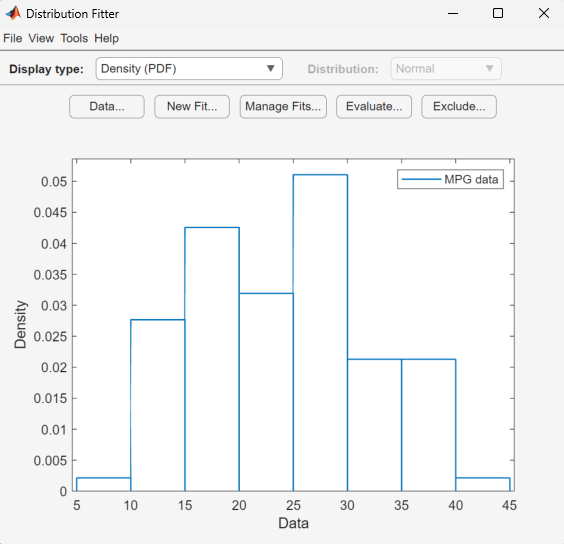Documentation

# Distribution Fitter

Fit probability distributions to data

## Description

The Distribution Fitter app interactively fits probability distributions to data imported from the MATLAB® workspace. You can choose from 22 built-in probability distributions or create your own custom distribution. The app displays plots of the fitted distribution superimposed on a histogram of the data. Available plots include probability density function (pdf), cumulative distribution function (cdf), probability plots, and survivor functions. You can export the fitted parameter values to the workspace as a probability distribution object, and use object functions to perform further analyses. For more information on working with these objects, see Working with Probability Distributions. For the programmatic work flow of the Distribution Fitter app, see `distributionFitter`.

### Required Products

• MATLAB

• Statistics and Machine Learning Toolbox™

Note: Distribution Fitter does not provide printing, custom distribution defining, or code generating functionality in MATLAB Online™.## Open the Distribution Fitter App

• MATLAB Toolstrip: On the Apps tab, under Math, Statistics and Optimization, click the app icon.

• MATLAB command prompt: Enter `distributionFitter`.

## Parameters

#### Data

Specify the data to import by selecting a variable from the drop-down list. If the variable is a matrix, the app imports the first column of the matrix by default. To select a different column or row of the matrix, click . Alternatively, you can enter any valid MATLAB expression in the field.

Specify the censoring data by selecting a variable from the drop-down list. If the variable is a matrix, the app imports the first column of the matrix by default. To select a different column or row of the matrix, click . This parameter is optional.

Specify the frequency data by selecting a variable from the drop-down list. If the variable is a matrix, the app imports the first column of the matrix by default. To select a different column or row of the matrix, click . This parameter is optional.

Specify a name for the data set as a character vector, or accept the default name.

Manage previously imported data sets. Click the data set of interest, then click the buttons below this pane to view the data (), set the bin rules (), rename the data set (), or delete the data set ().

Display a preview plot of the variable selected from the Data drop-down menu.

#### New fit

Specify a name for the fit or accept the default name.

Specify the data to fit by selecting a data set from the drop-down list.

Specify the distribution to fit by selecting a distribution name from the drop-down list.

Specify a rule to exclude some data values by selecting an exclusion rule from the drop-down list. To populate this drop-down list, you must first define exclusion rules by clicking in the main window of the app. This parameter is optional

#### Manage fits

Specify which fit or fits to plot in the main window by selecting the Plot check box next to each fit. Clear the Plot check box to remove a fit from the plot.

If you select Plot for a particular fit, you can select Conf bounds to display the confidence bounds for that fit on the plot in the main window. Clearing the Conf bounds check box removes the confidence intervals from the plot. The Distribution Fitter app displays confidence bounds only if the Display Type in the main window is set to Cumulative probability (CDF), Quantile (inverse CDF), Survivor function, or Cumulative hazard.

#### Evaluate

Select one or more fits from the list to evaluate.

Specify the type of probability function to evaluate from the drop-down list. Available probability functions include the probability density function (pdf), cumulative distribution function (cdf), quantile (inverse cdf), survival function, cumulative hazard, and hazard rate.

Specify a numeric vector of values at which to evaluate the function. If you specify Function as Quantile (inverse CDF), this field name changes to At p = and you enter a vector of probability values.

Select Compute confidence bounds to compute the confidence bounds for the selected fit. This check box is enabled only if you specify Function as Cumulative probability (CDF), Quantile (inverse CDF), Survivor function, or Cumulative hazard. This parameter is optional.

Specify the level at which to compute the confidence bounds. This check box is enabled only if you specify Function as Cumulative probability (CDF), Quantile (inverse CDF), Survivor function, or Cumulative hazard.

Select Plot function to display a plot of the distribution function, evaluated at the points that you enter in the At x = field, in a new window. This parameter is optional.

#### Exclude

Specify a name for the exclusion rule as a character vector.

Specify lower and upper limits for the data numerically.

Specify lower and upper limits for the data by selecting a variable from the Select data drop-down list and clicking Exclude graphically. An interactive plot opens in a new window, where you can add lower or upper limits by clicking and dragging a boundary on the plot.

Select an existing exclusion rule from the list. You can copy, view, rename, or delete exclusion rules by clicking the appropriate button.

## Programmatic Use

expand all

`distributionFitter` opens the Distribution Fitter app, or brings focus to the app if it is already open.

`distributionFitter(y)` opens the Distribution Fitter app populated with the data specified by the vector `y`.

`distributionFitter(y,cens)` uses the vector `cens` to specify whether the observation `y(j)` is censored, `(cens(j)==1)`, or observed exactly, `(cens(j)==0)`. If `cens` is omitted or empty, then no `y` values are censored.

`distributionFitter(y,cens,freq)` uses the vector `freq` to specify the frequency of each element contained in `y`. If `freq` is omitted or empty, then all values in `y` have a frequency of 1.

`distributionFitter(y,cens,freq,dsname)` creates a data set with the name `dsname` using the data vector, `y`, censoring indicator, `cens`, and frequency vector, `freq`. Specify `dsname` as a character vector or string scalar, for example, `'mydata'`.